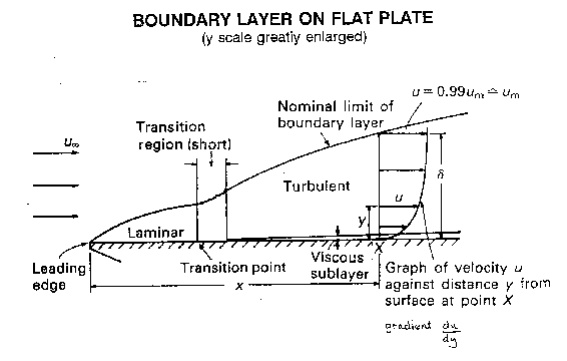Home | | Mechanics of Fluids | Mechanics Of Fluids- Boundary Layer

# Mechanics Of Fluids- Boundary LayerWhen a fluid flows over a stationary surface, e.g. the bed of a river, or the wall of a pipe, the fluidtouching the surface is brought to rest by the shear stress Ro at the wall. The velocity increases from thewall to a maximum in the main stream of the flow.

Boundary Layers

When a fluid flows over a stationary surface, e.g. the bed of a river, or the wall of a pipe, the fluidtouching the surface is brought to rest by the shear stress  to  at the wall. The velocity increases from thewall to a maximum in the main stream of the flow.Looking at this two-dimensionally we get the above velocity profile from the wall to the centre of the flow.

This profile doesn't just exit, it must build up gradually from the point where the fluid starts to flow past the surface - e.g. when it enters a pipe.

If we consider a flat plate in the middle of a fluid, we will look at the build up of the velocity profile as the fluid moves over the plate.

Upstream the velocity profile is uniform, (free stream flow) a long way downstream we have the velocity profile we have talked about above. This is the known as fully developed flow. But how do we get to that state?

This region, where there is a velocity profile in the flow due to the shear stress at the wall, we call the boundary layer. The stages of the formation of the boundary layer are shown in the figure below:We define the thickness of this boundary layer as the distance from the wall to the point where the velocity is 99% of the 'free stream' velocity, the velocity in the middle of the pipe or river.

boundary layer thickness, d = distance from wall to point where u = 0.99 umainstream

The value of d will increase with distance from the point where the fluid first starts to pass over the boundary - the flat plate in our example. It increases to a maximum in fully developed flow.

Correspondingly, the drag force D on the fluid due to shear stress to at the wall increases from zero at the start of the plate to a maximum in the fully developed flow region where it remains constant. We can calculate the magnitude of the drag force by using the momentum equation. But this complex and not necessary for this course.

Our interest in the boundary layer is that its presence greatly affects the flow through or round an object. So here we will examine some of the phenomena associated with the boundary layer and discuss why these occur.

Study Material, Lecturing Notes, Assignment, Reference, Wiki description explanation, brief detail
Civil - Mechanics Of Fluids - Boundary Layer : Mechanics Of Fluids- Boundary Layer |

Related Topics